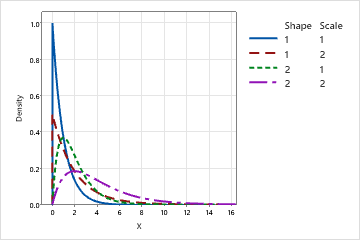# Gamma distribution

Use the gamma distribution to model positive data values that are skewed to the right and greater than 0. The gamma distribution is commonly used in reliability survival studies. For example, the gamma distribution can describe the time for an electrical component to fail. Most electrical components of a particular type will fail around the same time, but a few will take a long time to fail.

The gamma distribution is a continuous distribution that is defined by its shape and scale parameters. The 3-parameter gamma distribution is defined by its shape, scale, and threshold parameters. For example, in the following graph, the gamma distribution is defined by different shape and scale values when the threshold is set at 0.0. Notice that most values in a gamma distribution occur near each other, but some values trail into the upper tail.When the shape parameter is an integer, the gamma distribution is sometimes called an Erlang distribution. The Erlang distribution is commonly used in queuing theory applications.